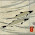## Wednesday, April 30, 2014

### Decision making trees and machine learning resources for R

I have recently come across Ricky Ho's blog "Pragmatic Programming Techniques", which seems to be excellent resource for all sorts of aspects regarding data exploration and predictive modelling. The post "Six steps in data science" provides a nice overview to some of the topics covered in the blog. For some reason, this blog does not seem to be listed on R-Bloggers (attn: Tal Galili!).

I was drawn to the page from my interest in understanding Classification and Regression Tree (CART) models, and quickly became amazed by the blog's nice review of some available methods. I was specifically looking for a example that uses Edgar Anderson's iris data set as I find it to be a very understandable example for this type of problem - i.e. Can we develop a model to predict the iris species based on it's morphological characteristics?

Below is a sample of just two methods that were presented using the rpart and randomForest packages. rpart is a referred to as a "Decision Tree" method, while randomForest is an example of a "Tree Ensemble" method. The blog explains many of the pros and cons for each method, and a further post show even further examples of predictive analytics, including Neural Network, Support Vector Machine, Naive Bayes and Nearest Neighbor approaches (all using the iris data set). I would love to know a bit more about the comparative predictive powers of each of these methods. For the meantime, the example below shows a cross-validation comparison of prediction accuracy for the rpart and randomForest methods using 100 permutations. Half the data set is used as the training set and the other half is used as the validation set.

The results show a slight improvement in accuracy for the randomForest method, especially for the species versicolor and virginica - which are more similar in morphology. This can be see in the degree of overlap in the plot of the first 2 principle components (explaining ~98% of the variance):

The setosa species is different enough that there is perfect (100%) accuracy in it's prediction. I'm looking forward to continuing with this comparison for the other methods as well.

Example code:

```### rpart
library(rpart)
set.seed(1)
perms <- 100
pred <- vector(mode="list", perms)
for(i in seq(perms)){
train <- sample(nrow(iris), nrow(iris)*0.5)
valid <- seq(nrow(iris))[-train]
iristrain <- iris[train,]
irisvalid <- iris[valid,]

model <- rpart(Species~., data=iristrain)
#Predict
prediction <- predict(model, newdata=irisvalid, type='class')
pred[[i]] <- table(prediction, irisvalid\$Species)
}
#accuracy overall
rpart.acc.all <- sapply(pred, FUN=function(x) sum(diag(x)) / sum(x))
mean(rpart.acc.all)
boxplot(rpart.acc.all, col="grey")

### accuracy by spp
rpart.acc.spp <- sapply(pred, FUN=function(x) diag(x) / colSums(x))
rowMeans(rpart.acc.spp)
boxplot(t(rpart.acc.spp), col=c(rgb(1,0.5,0.5), rgb(0.5,1,0.5), rgb(0.5,0.5,1)))

#randomForest
library(randomForest)
set.seed(1)
perms <- 100
pred <- vector(mode="list", perms)
for(i in seq(perms)){
train <- sample(nrow(iris), nrow(iris)*0.5)
valid <- seq(nrow(iris))[-train]
iristrain <- iris[train,]
irisvalid <- iris[valid,]

model <- randomForest(Species~., data=iristrain, nTree=500)
#Predict using the forest
prediction <- predict(model, newdata=irisvalid, type='class')
pred[[i]] <- table(prediction, irisvalid\$Species)
#importance(model)
}
#accuracy overall
randomForest.acc.all <- sapply(pred, FUN=function(x) sum(diag(x)) / sum(x))
mean(randomForest.acc.all)
boxplot(randomForest.acc.all, col="grey")

#accuracy by spp
randomForest.acc.spp <- sapply(pred, FUN=function(x) diag(x) / colSums(x))
rowMeans(randomForest.acc.spp)
boxplot(t(randomForest.acc.spp), col=c(rgb(1,0.5,0.5), rgb(0.5,1,0.5), rgb(0.5,0.5,1)))

### plot
png("CART_model_accuracy.png", width=7, height=5, units="in", res=400, type="cairo")
par(mar=c(8,4,2,1))
comb.acc.spp <- rbind(rpart.acc.spp, randomForest.acc.spp) * 100
comb.acc.spp <- comb.acc.spp[c(1,4,2,5,3,6),]
boxplot(
t(rbind(comb.acc.spp)),
col=c(
rgb(1,0.5,0.5), rgb(1,0.7,0.7),
rgb(0.5,1,0.5), rgb(0.7,1,0.7),
rgb(0.5,0.5,1), rgb(0.7,0.7,1)
),
names=paste0(rep(levels(iris\$Species), each=2), c("\nrpart", "\nrandomForest")),
las=2,
ylab="CART model accuracy [%]"
)
mtext("Species classification - Edgar Anderson's Iris Data", side=3, line=0.5)
dev.off()

png("iris_pca.png", units="in", height=6, width=6, res=400)
par(mar=c(4,4,1,1))
pca <- prcomp(iris[,1:4])
plot(pca\$x[,1:2], col=c(2:4)[iris\$Species])
sc <- 2
arrows(0,0, x1=pca\$rotation[,1]*pca\$sdev*sc, y1=pca\$rotation[,2]*pca\$sdev*sc)
text(x=pca\$rotation[,1]*pca\$sdev*sc, y=pca\$rotation[,2]*pca\$sdev*sc, labels=rownames(pca\$rotation), pos=1)
legend("topright", legend=levels(iris\$Species), col=2:4, pch=1)
dev.off()```
Created by Pretty R at inside-R.org

1.did I do something wrong?

> library(rpart)
> set.seed(1)
> perms <- 100
> pred <- vector(mode="list", perms)
> for(i in seq(perms)){
+ train <- sample(nrow(iris), nrow(iris)*0.5)
+ valid <- seq(nrow(iris))[-train]
+ iristrain <- iris[train,]
+ irisvalid <- iris[valid,]
+
+ model <- rpart(Species~., data=iristrain)
+ #Predict
+ prediction <- predict(model, newdata=irisvalid, type='class')
+ pred[[i]] <- table(prediction, iristest\$Species)
+ }
Error in table(prediction, iristest\$Species) :

1.Hi Rick - That's embarrassing. I had changed a variable name at some point. I have corrected the code now - thanks for pointing that out!

2.Thanks for the great post! This inspired me to investigate CART models a bit more. I found a set of course notes from Cosma Shlizi quite helpful, and thought I'd pass them along. They're basically a first introduction to prediction trees, but I thought you might be interested nonetheless:

http://www.stat.cmu.edu/~cshalizi/350/lectures/22/lecture-22.pdf

1.Thanks for your comment, and for passing along this resource Mark. Like your blog by the way. Cheers, Marc